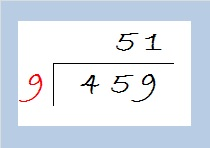# 459 and Level 2

459 is the hypotenuse of this Pythagorean triple: 216-405-459.

What is the greatest common factor of those three numbers?

The GCF has to be a factor of the smallest number, 216, and it has to be an odd number because at least one of the other numbers is odd. Let’s factor out the even factors of 216 to find its greatest odd factor:

• 216 can be evenly divided by 4 because the last two digits form a multiple of 4.
• It can also be evenly divided by 8 because 16 is a multiple of 8 and the 3rd from the right digit is even.
• 216 ÷ 8 = 27.
• Check to see if the other two numbers in the triple are divisible by 27, and you will see that 27 is the GCF of 216-405-459.To solve this puzzle ask yourself:

What is a common factor of 6, 14, 10, and 16? What about 3, 18, 6, and 30? And what is a common factor of 9, 36, 45, 63, 54, 90, 72? In each case, the common factor has to be a factor of the smallest number on the list, and if any of the numbers on the list are odd, it has to be an odd number. (For level 1 and level 2 puzzles, that factor will oftrn be the greatest common factor of all the numbers in a particular row or column.)

Print the puzzles or type the solution on this excel file:  10 Factors 2015-04-13

—————————————————————————————————

459 cannot be evenly divided by 100 or by 4, but it is divisible by 9. To find it square root, let’s first divide 459 by 9:The quotient, 51, is small enough that we can recognize that it cannot be evenly divided by any square number less than it. Thus we take the square root of everything on the outside of the cake and get √459 = (√9)(√51) = 3√51.

• 459 is a composite number.
• Prime factorization: 459 = 3 x 3 x 3 x 17, which can be written 459 = (3^3) x 17
• The exponents in the prime factorization are 3 and 1. Adding one to each and multiplying we get (3 + 1)(1 + 1) = 4 x 2 = 8. Therefore 459 has exactly 8 factors.
• Factors of 459: 1, 3, 9, 17, 27, 51, 153, 459
• Factor pairs: 459 = 1 x 459, 3 x 153, 9 x 51, or 17 x 27
• Taking the factor pair with the largest square number factor, we get √459 = (√9)(√51) = 3√51 ≈ 21.4243—————————————————————————————————This site uses Akismet to reduce spam. Learn how your comment data is processed.